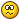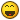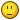## Recommended Posts

Hola Bueno como dice el post quiero una quest que cuando suva al Nivel 40 Me Suva automaticamente al nivel 130

##### Share on other sites

Lo que creo que necesitas es: dif, quest, y saberPor qué nunca he visto esa función##### Share on other sites

Lo que creo que necesitas es: dif, quest, y saberPor qué nunca he visto esa funciónEmm no creo que sea diff porque vi un post hace tiempos una quest que si suvia al Level 200 suvia automaticamente al Nivel 250

##### Share on other sites

Emm no creo que sea diff porque vi un post hace tiempos una quest que si suvia al Level 200 suvia automaticamente al Nivel 250

La hubieras copiadoBueno algo parecido sería esto:

Debes iniciar sesión para ver el contenido del enlace en esta publicación.

Pero lo malo, es que tendríais que copiarla muchas veces##### Share on other sites

La hubieras copiadoBueno algo parecido sería esto:

Debes iniciar sesión para ver el contenido del enlace en esta publicación.

Pero lo malo, es que tendríais que copiarla muchas vecesNo es esa men aver era una quest que cuando uno suvia al nivel 40 te suvia al istante al level maximo

##### Share on other sitesOstia es que acaso no leéis lo que escribí? Es algo parecido, pero tendríais que poner muchas veces la función.

##### Share on other sites

debes usar un while##### Share on other sites

:/ nose es una pendeja quest que no la incuentro pero es una quest yo se que si porque una ves la utilize pero nose si todiavia esta

##### Share on other sites

while pc.get_level() <= 130

pc.give_exp2(100000000000000)

end

##### Share on other sites

while pc.get_level() <= 130

pc.give_exp2(100000000000000)

end

¿?

##### Share on other sitestienes la parte encarga de subirte de lv solo pones la estructura basica de quest y ya##### Share on other sitestienes la parte encarga de subirte de lv solo pones la estructura basica de quest y yaPodrias hablarme por mensaje

¿

##### Share on other sites

Algo así creoPor lo que entiendo de Raiser claroquest metin2zone begin
state start begin
while pc.get_level() <= 130 do
pc.give_exp2(1000000000)
end
end
end
end

##### Share on other sites

Algo así creoPor lo que entiendo de Raiser claroquest metin2zone begin

state start begin

while pc.get_level() <= 130 do

pc.give_exp2(1000000000)

end

end

end

end

ases un login o subes de nivel te sube al 130 ee :v sin importar tu nivel  1 o 2

when xx or xx with pc.get_level() >= xxx begin

##### Share on other sites

no se mucho de querys pero a lo poco que e visto se me ocurre esto

```quest nivelmaximo begin
state start beginwhen login or levelup with pc.level >= 40
command("level "..personaje().." 130")

```

##### Share on other sites

ases un login o subes de nivel te sube al 130 ee :v sin importar tu nivel  1 o 2

when xx or xx with pc.get_level() >= xxx begin

Bueno pues así ...

quest metin2zone begin

state start begin

when login with pc.get_level() == 40 begin

while pc.get_level() <= 130 do

pc.give_exp2(1000000000)

end

end

end

end

##### Share on other sites

no se mucho de querys pero a lo poco que e visto se me ocurre esto

```quest nivelmaximo begin
state start beginwhen login or levelup with pc.level >= 40
command("level "..personaje().." 130")
```

Primero: es lua(quest), no querySegundo: al poner "command" solo servirá para gm's##### Share on other sites
```quest lv_start begin
state start begin
pc.get_level()== 40
while pc.get_level() <== 130 do
pc.give_exp (25000000000)
end
end
end
end
```

No tengo ni zorra idea pero creo que es algo asi xD no me agais mucho caso##### Share on other sites

pc.set_level(130)

eso es lo que buscas

##### Share on other sites
```pc.set_level(130)

eso es lo que buscas
```

Deberias hacer la quest ya que la mayoria de aqui no sabemos ni zorra de LUA xD ademas tu tardas dos minutos

##### Share on other sites
```pc.set_level(130)

eso es lo que buscas
```

Yo veo clarísimo que hagas un aporte de esa función##### Share on other sites
```pc.set_level(130)

eso es lo que buscas
```

LOL me olvide de esa funcion D:

##### Share on other sites

Bueno yo te echo esta a partir de una de pentagrama, lo que hace es que te pide un objeto y con ese te sube al 100 :v, puedes hacer que a nivel 40 reciba ese objeto automáticamente.

--Quest by PENTAGRAMA--

--Solo para Metin2Zone--
quest SubirNivel begin
state start begin
when 30327.use begin   --##Item ID

local e= pc.get_next_exp()
pc.give_exp2(e)

local e= pc.get_next_exp()
pc.give_exp2(e)

local e= pc.get_next_exp()
pc.give_exp2(e)

local e= pc.get_next_exp()
pc.give_exp2(e)

local e= pc.get_next_exp()
pc.give_exp2(e)

local e= pc.get_next_exp()
pc.give_exp2(e)

local e= pc.get_next_exp()
pc.give_exp2(e)

local e= pc.get_next_exp()
pc.give_exp2(e)

local e= pc.get_next_exp()
pc.give_exp2(e)

local e= pc.get_next_exp()
pc.give_exp2(e)

local e= pc.get_next_exp()
pc.give_exp2(e)

local e= pc.get_next_exp()
pc.give_exp2(e)

local e= pc.get_next_exp()
pc.give_exp2(e)

local e= pc.get_next_exp()
pc.give_exp2(e)

local e= pc.get_next_exp()
pc.give_exp2(e)

local e= pc.get_next_exp()
pc.give_exp2(e)

local e= pc.get_next_exp()
pc.give_exp2(e)

local e= pc.get_next_exp()
pc.give_exp2(e)

local e= pc.get_next_exp()
pc.give_exp2(e)

local e= pc.get_next_exp()
pc.give_exp2(e)

local e= pc.get_next_exp()
pc.give_exp2(e)

local e= pc.get_next_exp()
pc.give_exp2(e)

local e= pc.get_next_exp()
pc.give_exp2(e)

local e= pc.get_next_exp()
pc.give_exp2(e)

local e= pc.get_next_exp()
pc.give_exp2(e)

local e= pc.get_next_exp()
pc.give_exp2(e)

local e= pc.get_next_exp()
pc.give_exp2(e)

local e= pc.get_next_exp()
pc.give_exp2(e)

local e= pc.get_next_exp()
pc.give_exp2(e)

local e= pc.get_next_exp()
pc.give_exp2(e)

local e= pc.get_next_exp()
pc.give_exp2(e)

local e= pc.get_next_exp()
pc.give_exp2(e)

local e= pc.get_next_exp()
pc.give_exp2(e)

local e= pc.get_next_exp()
pc.give_exp2(e)

local e= pc.get_next_exp()
pc.give_exp2(e)

local e= pc.get_next_exp()
pc.give_exp2(e)

local e= pc.get_next_exp()
pc.give_exp2(e)

local e= pc.get_next_exp()
pc.give_exp2(e)

local e= pc.get_next_exp()
pc.give_exp2(e)

local e= pc.get_next_exp()
pc.give_exp2(e)

local e= pc.get_next_exp()
pc.give_exp2(e)

local e= pc.get_next_exp()
pc.give_exp2(e)

local e= pc.get_next_exp()
pc.give_exp2(e)

local e= pc.get_next_exp()
pc.give_exp2(e)

local e= pc.get_next_exp()
pc.give_exp2(e)

local e= pc.get_next_exp()
pc.give_exp2(e)

local e= pc.get_next_exp()
pc.give_exp2(e)

local e= pc.get_next_exp()
pc.give_exp2(e)

local e= pc.get_next_exp()
pc.give_exp2(e)

local e= pc.get_next_exp()
pc.give_exp2(e)

local e= pc.get_next_exp()
pc.give_exp2(e)

local e= pc.get_next_exp()
pc.give_exp2(e)

local e= pc.get_next_exp()
pc.give_exp2(e)

local e= pc.get_next_exp()
pc.give_exp2(e)

local e= pc.get_next_exp()
pc.give_exp2(e)

local e= pc.get_next_exp()
pc.give_exp2(e)

local e= pc.get_next_exp()
pc.give_exp2(e)

local e= pc.get_next_exp()
pc.give_exp2(e)

local e= pc.get_next_exp()
pc.give_exp2(e)

local e= pc.get_next_exp()
pc.give_exp2(e)

local e= pc.get_next_exp()
pc.give_exp2(e)

local e= pc.get_next_exp()
pc.give_exp2(e)

local e= pc.get_next_exp()
pc.give_exp2(e)

local e= pc.get_next_exp()
pc.give_exp2(e)

local e= pc.get_next_exp()
pc.give_exp2(e)

local e= pc.get_next_exp()
pc.give_exp2(e)

local e= pc.get_next_exp()
pc.give_exp2(e)

local e= pc.get_next_exp()
pc.give_exp2(e)

local e= pc.get_next_exp()
pc.give_exp2(e)

local e= pc.get_next_exp()
pc.give_exp2(e)

local e= pc.get_next_exp()
pc.give_exp2(e)

local e= pc.get_next_exp()
pc.give_exp2(e)

local e= pc.get_next_exp()
pc.give_exp2(e)

local e= pc.get_next_exp()
pc.give_exp2(e)

local e= pc.get_next_exp()
pc.give_exp2(e)

local e= pc.get_next_exp()
pc.give_exp2(e)

local e= pc.get_next_exp()
pc.give_exp2(e)

local e= pc.get_next_exp()
pc.give_exp2(e)

local e= pc.get_next_exp()
pc.give_exp2(e)

local e= pc.get_next_exp()
pc.give_exp2(e)

local e= pc.get_next_exp()
pc.give_exp2(e)

local e= pc.get_next_exp()
pc.give_exp2(e)

local e= pc.get_next_exp()
pc.give_exp2(e)

local e= pc.get_next_exp()
pc.give_exp2(e)

local e= pc.get_next_exp()
pc.give_exp2(e)

local e= pc.get_next_exp()
pc.give_exp2(e)

local e= pc.get_next_exp()
pc.give_exp2(e)

local e= pc.get_next_exp()
pc.give_exp2(e)

local e= pc.get_next_exp()
pc.give_exp2(e)

local e= pc.get_next_exp()
pc.give_exp2(e)

local e= pc.get_next_exp()
pc.give_exp2(e)

local e= pc.get_next_exp()
pc.give_exp2(e)

local e= pc.get_next_exp()
pc.give_exp2(e)

local e= pc.get_next_exp()
pc.give_exp2(e)

pc.remove_item(30327, 1) --##Item borrarlo (el ID, cuantos)
chat(pc.get_name()..", ha subido al 130.")--editas lo que dira
end
end
end

xd creo que leí que para lo que tu quieres se necesita un diff

##### Share on other sites

Bueno yo te echo esta a partir de una de pentagrama, lo que hace es que te pide un objeto y con ese te sube al 100 :v, puedes hacer que a nivel 40 reciba ese objeto automáticamente.

--Quest by PENTAGRAMA--

--Solo para Metin2Zone--
quest SubirNivel begin
state start begin
when 30327.use begin   --##Item ID

local e= pc.get_next_exp()
pc.give_exp2(e)

local e= pc.get_next_exp()
pc.give_exp2(e)

local e= pc.get_next_exp()
pc.give_exp2(e)

local e= pc.get_next_exp()
pc.give_exp2(e)

local e= pc.get_next_exp()
pc.give_exp2(e)

local e= pc.get_next_exp()
pc.give_exp2(e)

local e= pc.get_next_exp()
pc.give_exp2(e)

local e= pc.get_next_exp()
pc.give_exp2(e)

local e= pc.get_next_exp()
pc.give_exp2(e)

local e= pc.get_next_exp()
pc.give_exp2(e)

local e= pc.get_next_exp()
pc.give_exp2(e)

local e= pc.get_next_exp()
pc.give_exp2(e)

local e= pc.get_next_exp()
pc.give_exp2(e)

local e= pc.get_next_exp()
pc.give_exp2(e)

local e= pc.get_next_exp()
pc.give_exp2(e)

local e= pc.get_next_exp()
pc.give_exp2(e)

local e= pc.get_next_exp()
pc.give_exp2(e)

local e= pc.get_next_exp()
pc.give_exp2(e)

local e= pc.get_next_exp()
pc.give_exp2(e)

local e= pc.get_next_exp()
pc.give_exp2(e)

local e= pc.get_next_exp()
pc.give_exp2(e)

local e= pc.get_next_exp()
pc.give_exp2(e)

local e= pc.get_next_exp()
pc.give_exp2(e)

local e= pc.get_next_exp()
pc.give_exp2(e)

local e= pc.get_next_exp()
pc.give_exp2(e)

local e= pc.get_next_exp()
pc.give_exp2(e)

local e= pc.get_next_exp()
pc.give_exp2(e)

local e= pc.get_next_exp()
pc.give_exp2(e)

local e= pc.get_next_exp()
pc.give_exp2(e)

local e= pc.get_next_exp()
pc.give_exp2(e)

local e= pc.get_next_exp()
pc.give_exp2(e)

local e= pc.get_next_exp()
pc.give_exp2(e)

local e= pc.get_next_exp()
pc.give_exp2(e)

local e= pc.get_next_exp()
pc.give_exp2(e)

local e= pc.get_next_exp()
pc.give_exp2(e)

local e= pc.get_next_exp()
pc.give_exp2(e)

local e= pc.get_next_exp()
pc.give_exp2(e)

local e= pc.get_next_exp()
pc.give_exp2(e)

local e= pc.get_next_exp()
pc.give_exp2(e)

local e= pc.get_next_exp()
pc.give_exp2(e)

local e= pc.get_next_exp()
pc.give_exp2(e)

local e= pc.get_next_exp()
pc.give_exp2(e)

local e= pc.get_next_exp()
pc.give_exp2(e)

local e= pc.get_next_exp()
pc.give_exp2(e)

local e= pc.get_next_exp()
pc.give_exp2(e)

local e= pc.get_next_exp()
pc.give_exp2(e)

local e= pc.get_next_exp()
pc.give_exp2(e)

local e= pc.get_next_exp()
pc.give_exp2(e)

local e= pc.get_next_exp()
pc.give_exp2(e)

local e= pc.get_next_exp()
pc.give_exp2(e)

local e= pc.get_next_exp()
pc.give_exp2(e)

local e= pc.get_next_exp()
pc.give_exp2(e)

local e= pc.get_next_exp()
pc.give_exp2(e)

local e= pc.get_next_exp()
pc.give_exp2(e)

local e= pc.get_next_exp()
pc.give_exp2(e)

local e= pc.get_next_exp()
pc.give_exp2(e)

local e= pc.get_next_exp()
pc.give_exp2(e)

local e= pc.get_next_exp()
pc.give_exp2(e)

local e= pc.get_next_exp()
pc.give_exp2(e)

local e= pc.get_next_exp()
pc.give_exp2(e)

local e= pc.get_next_exp()
pc.give_exp2(e)

local e= pc.get_next_exp()
pc.give_exp2(e)

local e= pc.get_next_exp()
pc.give_exp2(e)

local e= pc.get_next_exp()
pc.give_exp2(e)

local e= pc.get_next_exp()
pc.give_exp2(e)

local e= pc.get_next_exp()
pc.give_exp2(e)

local e= pc.get_next_exp()
pc.give_exp2(e)

local e= pc.get_next_exp()
pc.give_exp2(e)

local e= pc.get_next_exp()
pc.give_exp2(e)

local e= pc.get_next_exp()
pc.give_exp2(e)

local e= pc.get_next_exp()
pc.give_exp2(e)

local e= pc.get_next_exp()
pc.give_exp2(e)

local e= pc.get_next_exp()
pc.give_exp2(e)

local e= pc.get_next_exp()
pc.give_exp2(e)

local e= pc.get_next_exp()
pc.give_exp2(e)

local e= pc.get_next_exp()
pc.give_exp2(e)

local e= pc.get_next_exp()
pc.give_exp2(e)

local e= pc.get_next_exp()
pc.give_exp2(e)

local e= pc.get_next_exp()
pc.give_exp2(e)

local e= pc.get_next_exp()
pc.give_exp2(e)

local e= pc.get_next_exp()
pc.give_exp2(e)

local e= pc.get_next_exp()
pc.give_exp2(e)

local e= pc.get_next_exp()
pc.give_exp2(e)

local e= pc.get_next_exp()
pc.give_exp2(e)

local e= pc.get_next_exp()
pc.give_exp2(e)

local e= pc.get_next_exp()
pc.give_exp2(e)

local e= pc.get_next_exp()
pc.give_exp2(e)

local e= pc.get_next_exp()
pc.give_exp2(e)

local e= pc.get_next_exp()
pc.give_exp2(e)

local e= pc.get_next_exp()
pc.give_exp2(e)

local e= pc.get_next_exp()
pc.give_exp2(e)

local e= pc.get_next_exp()
pc.give_exp2(e)

local e= pc.get_next_exp()
pc.give_exp2(e)

local e= pc.get_next_exp()
pc.give_exp2(e)

pc.remove_item(30327, 1) --##Item borrarlo (el ID, cuantos)
chat(pc.get_name()..", ha subido al 130.")--editas lo que dira
end
end
end

xd creo que leí que para lo que tu quieres se necesita un diff

Un bucle for sería mejor para no repetir tanto esto: xD

local e= pc.get_next_exp()
pc.give_exp2(e)

No entiendo mucho LUA pero sería algo así, supongo.

```for i=1, 100 do
local e = pc.get_next_exp()
pc.give_exp2(e)
end```

##### Share on other sites

Un bucle for sería mejor para no repetir tanto esto: xD

local e= pc.get_next_exp()
pc.give_exp2(e)

No entiendo mucho LUA pero sería algo así, supongo.

```for i=1, 100 do
local e = pc.get_next_exp()
pc.give_exp2(e)
end```

Hahaha tiene razón, es que tampoco se mucho de eso,  pero lo malo es que no consigo que suba al 130 a pesar de ponerle mas, se queda en 100, y el ítem solo funciona 1 vez, lo que el quiere creo que se necesita un diff igual que si quieres que te baje de nivel